# MAT 4830 Mathematical Modeling Section 1 3 Conditional

• Slides: 31
Download presentation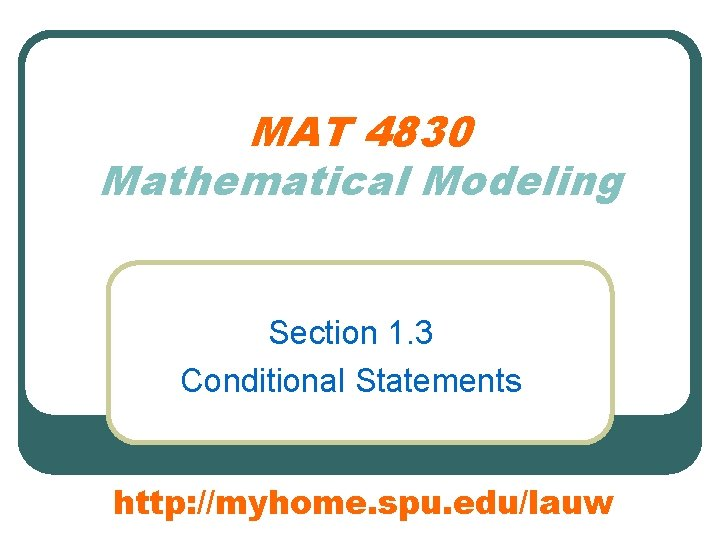MAT 4830 Mathematical Modeling Section 1. 3 Conditional Statements http: //myhome. spu. edu/lauw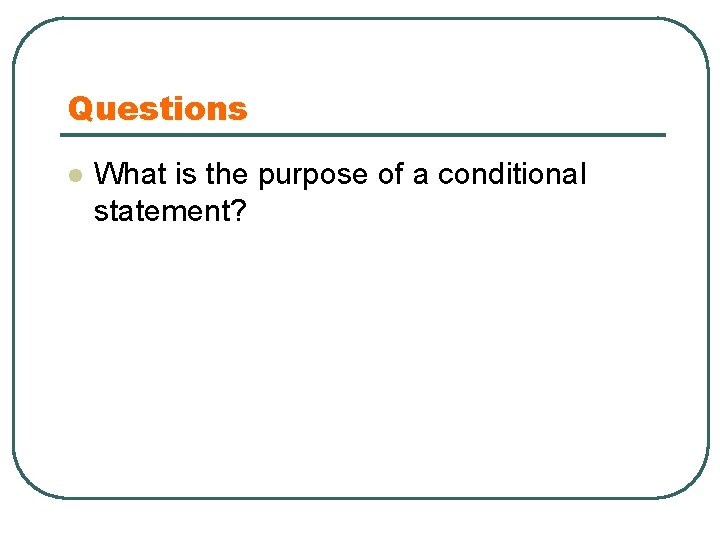Questions l What is the purpose of a conditional statement?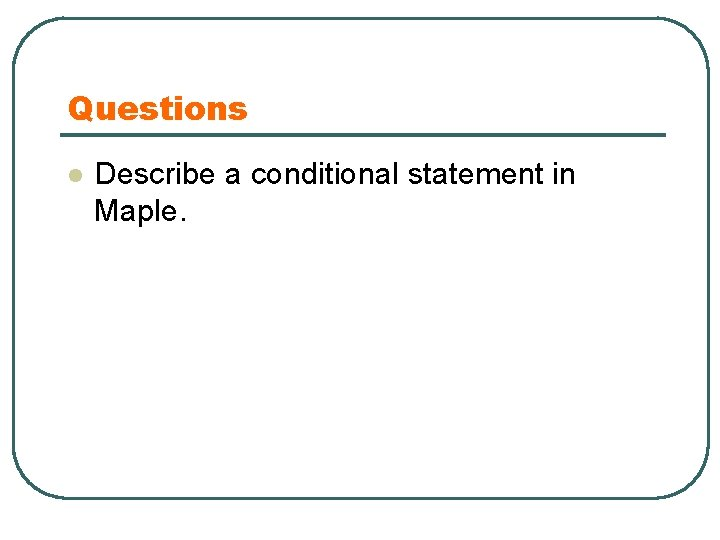Questions l Describe a conditional statement in Maple.Starting Next Monday l l You will be writing codes without an example that you can just modify on. You need to be able to write complete Maple programs on your own. No two should sit next to each other today. Learn to debug your own program is an important learning process.Preview l l l Review Poisson Distribution Introduces the conditional statements Allow the flow of control to branch into two or more sections of codes based on the truth values of a control expressions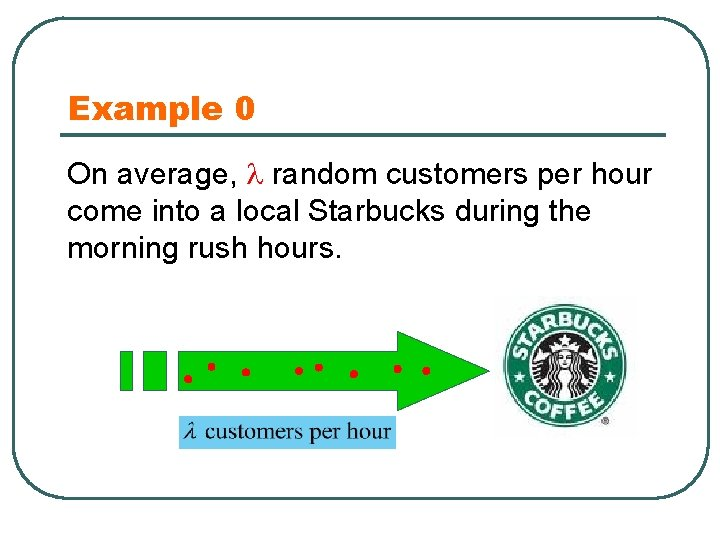Example 0 On average, random customers per hour come into a local Starbucks during the morning rush hours.Example 0 l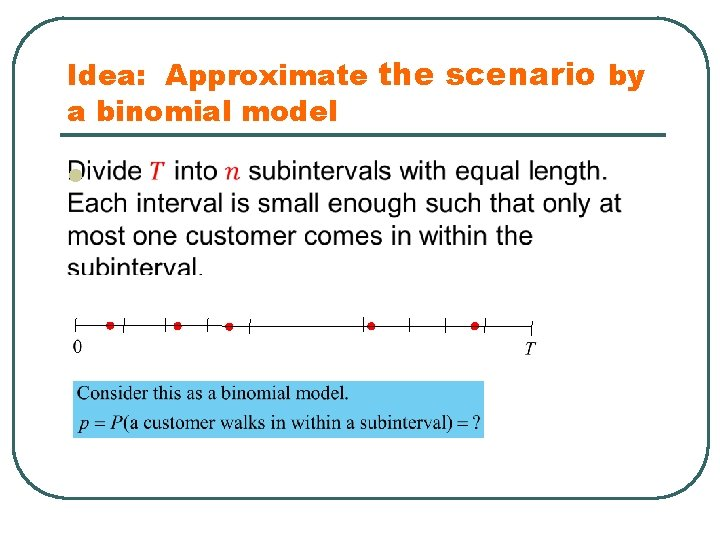Idea: Approximate the scenario by a binomial model l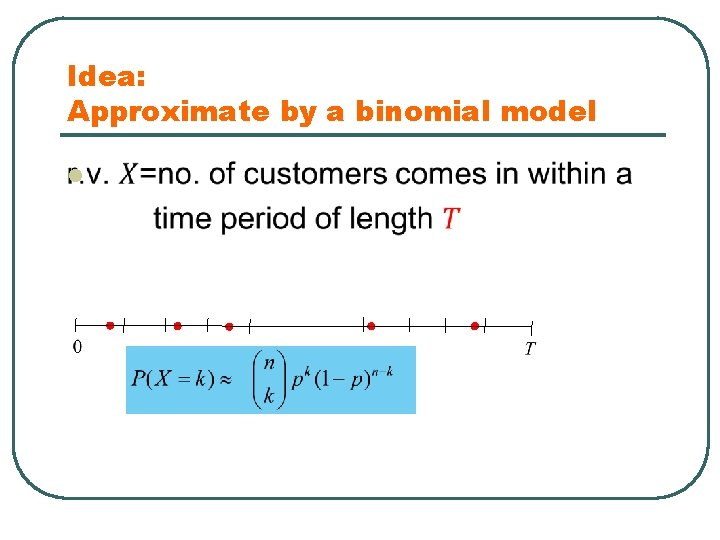Idea: Approximate by a binomial model lIdea: Approximate by a binomial model lTheorem 1Why? Calculus Formula:Why?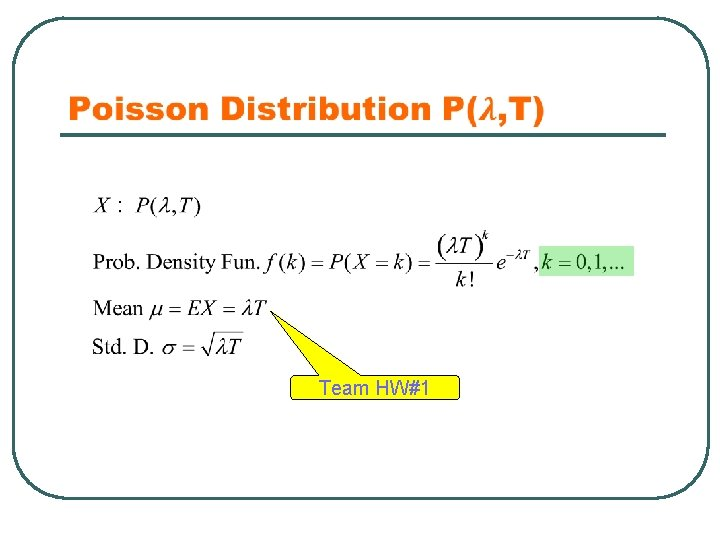Team HW#1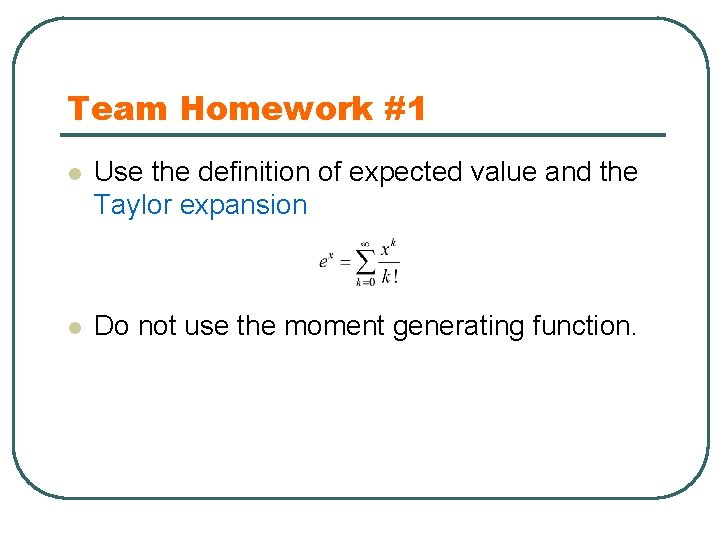Team Homework #1 l Use the definition of expected value and the Taylor expansion l Do not use the moment generating function.Poisson Distribution lTeam Homework #2 A newsboy sells newspapers outside Grand Central Station. He has on average 100 customers per day. He buys papers for 50 cents each, sells them for 75 cents each, but cannot return unsold papers for a refund. How many papers should he buy? To maximize the expected profit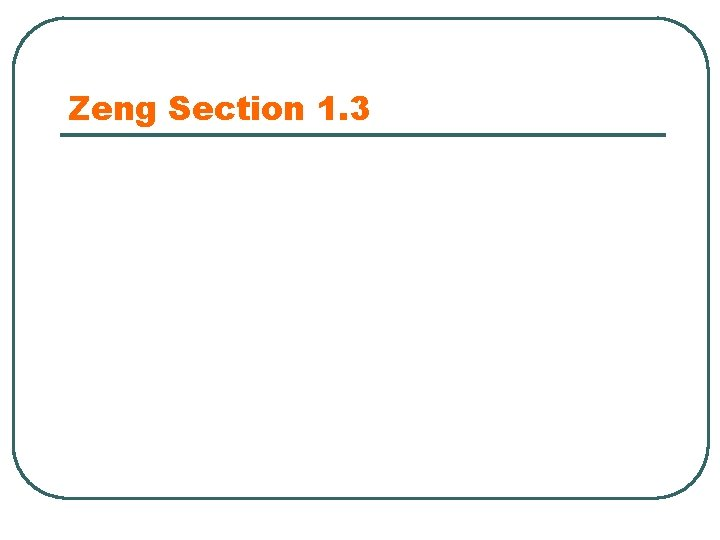Zeng Section 1. 3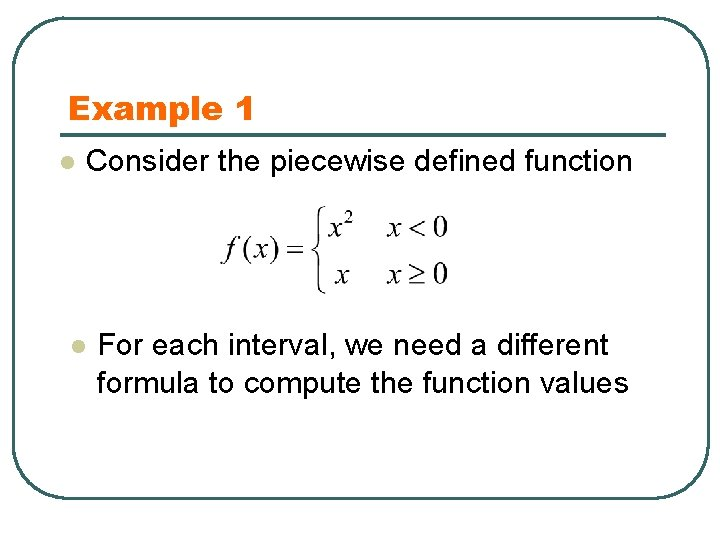Example 1 l Consider the piecewise defined function l For each interval, we need a different formula to compute the function valuesExample 1 l Consider the piecewise defined function For each interval, we need a different formula to compute the function values Q: Input=? , Output=? lExample 1 Version 1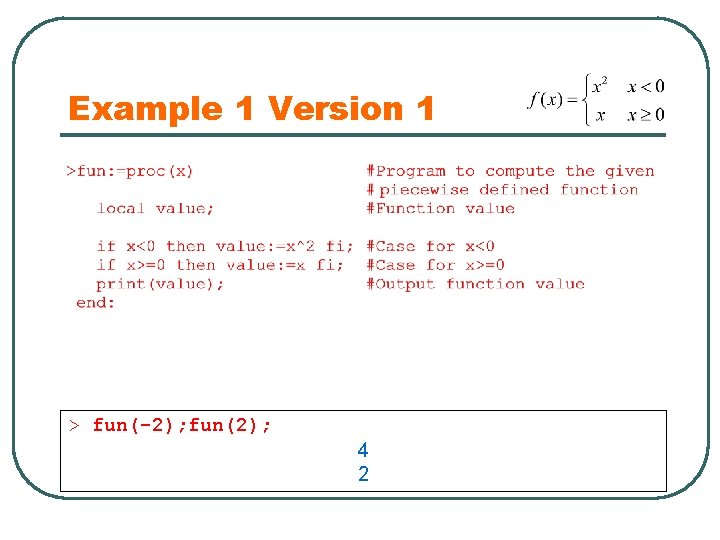Example 1 Version 1 > fun(-2); fun(2); 4 2Structure of the if-block Statements executed only if the condition is met. Otherwise, the statements will be skipped:Example 1 Version 2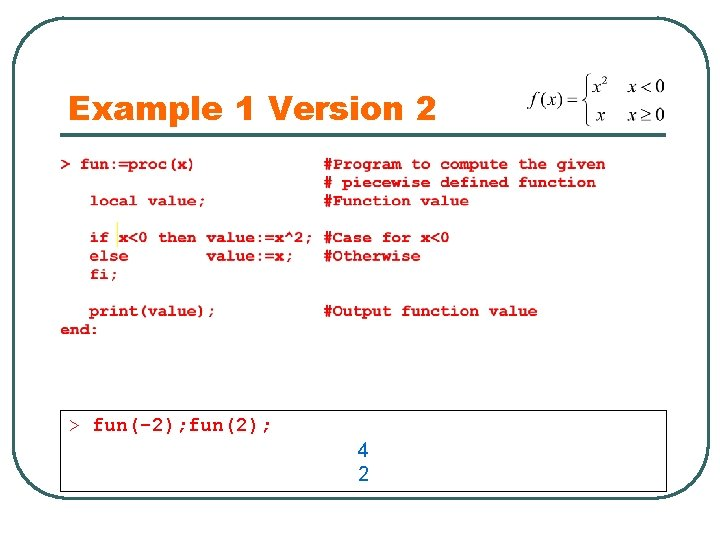Example 1 Version 2 > fun(-2); fun(2); 4 2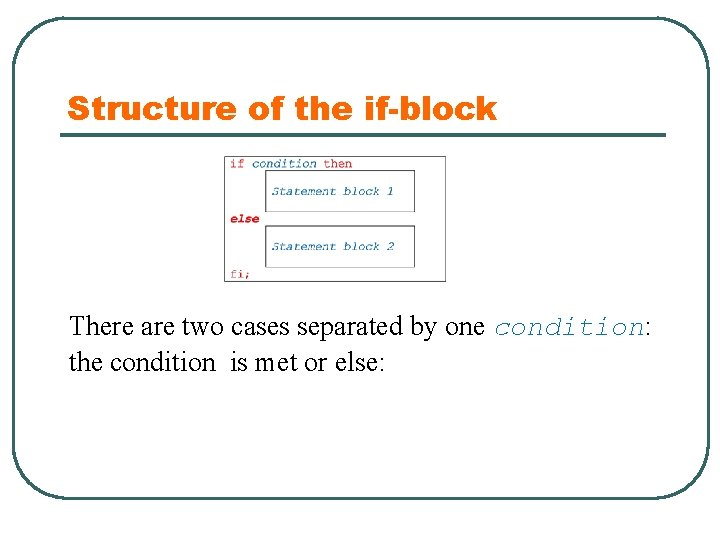Structure of the if-block There are two cases separated by one condition: the condition is met or else: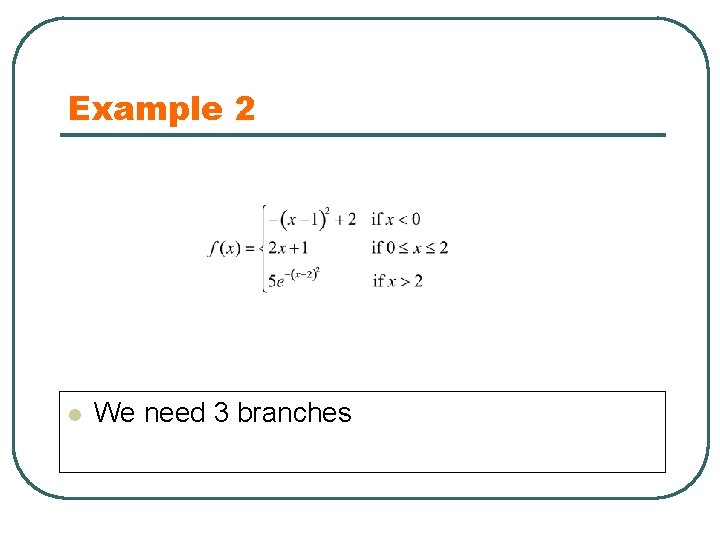Example 2 l We need 3 branchesExample 2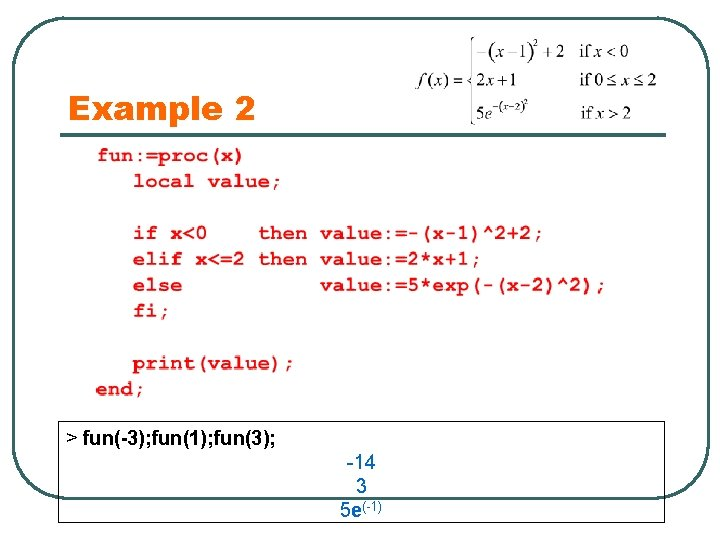Example 2 > fun(-3); fun(1); fun(3); -14 3 5 e(-1)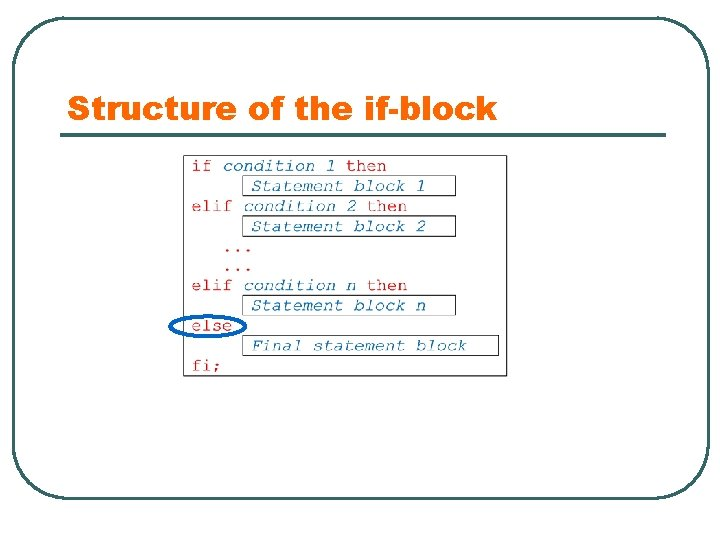Structure of the if-block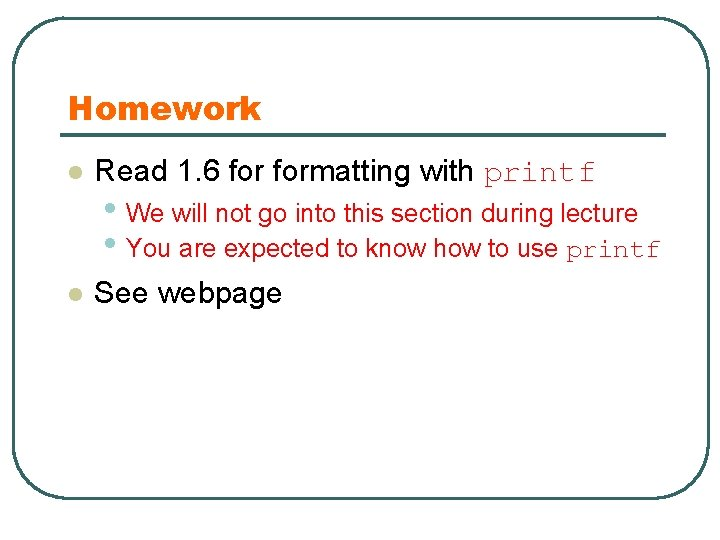Homework l Read 1. 6 formatting with printf • We will not go into this section during lecture • You are expected to know how to use printf l See webpage